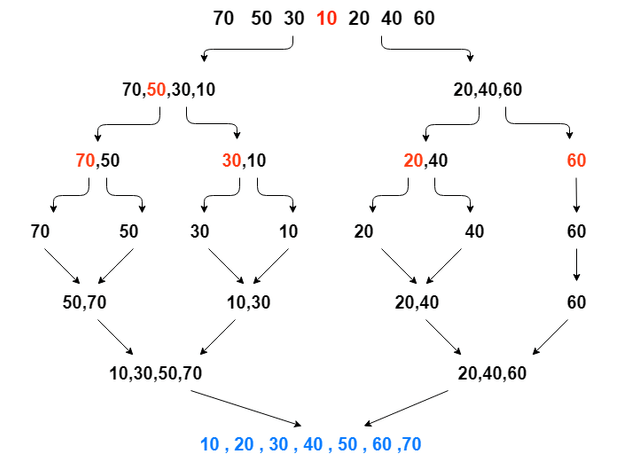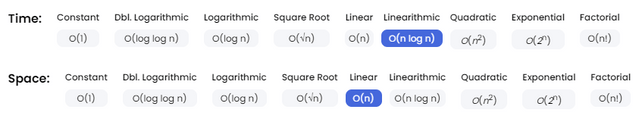# How does Merge Sort work?

Technology CommunityCategory: Divide & ConquerHow does Merge Sort work?

Merge sort is a divide-and-conquercomparison-based sorting algorithm based on the idea of breaking down a list into several sub-lists until each sublist consists of a single element and merging those sublists in a manner that results into a sorted list. Most implementations produce a stable sort, which means that the order of equal elements is the same in the input and output. Can be used as external sorting (when the data to be sorted is too large to fit into memory).There are two methods for implementing a Merge Sort algorithm:

• The Top-Down (recursive) approach. Given an array of size N, the algorithm recursively breaks the array in half and then merges the results together.
• The Bottom-Up (iterative) approach. Rather than breaking the overall array into distinct pieces, bottum-up mergesort loops over the array using intervals of varying sizes. Each interval is sorted and merged together; subsequent loops over the array have larger intervals, effectively merging our previously sorted (smaller) intervals together.
Complexity AnalysisThe list of size n is divided into a max of log n parts (the height of the conquer step tree is log n + 1 for a given n), and the merging of all sublists into a single list takes O(n) time, the worst case run time of this algorithm is O(n log n).Space complexity is always Ω(n) as you have to store the elements somewhere. Additional space complexity can be O(n) in an implementation using arrays and O(1) in linked list implementations. In practice implementations using lists need additional space for list pointers, so unless you already have the list in memory it shouldn’t matter.

Implementation

The Top-Down (recursive) approach

function mergeSort(arr) {  if (arr.length < 2) {    return arr;  }    let midpoint = Math.floor(arr.length / 2);  let leftHalf = arr.slice(0, midpoint); //slice an array of left-half items  let rightHalf = arr.slice(midpoint); //slice an array of right-half items    //recurse into each half until arrays are 1 item each  //then merge() the sorted arrays on each layer of the stack  return merge(mergeSort(leftHalf), mergeSort(rightHalf));}//first time this merge() gets called, the arr's are both 1 element long//on each layer of the stack the arrs will be doubled and always be sortedfunction merge(arrA, arrB) {  let a = 0;  let b = 0;  let result = [];    while (a < arrA.length && b < arrB.length) {    if (arrA[a] < arrB[b]) {      result.push(arrA[a]);      a++;    } else {      result.push(arrB[b]);      b++;    }  }  //using slice to concat remaining items left in either arrA or arrB  return result.concat(arrA.slice(a)).concat(arrB.slice(b)); }//usage:mergeSort([4,2,8,3,6,9,1]); //=> [1,2,3,4,6,8,9]

The Bottom-Up (iterative) approach

function mergeSort(arr) {    var sorted = arr.slice(),        n = sorted.length,        buffer = new Array(n);    for (var size = 1; size < n; size *= 2) {        for (var leftStart = 0; leftStart < n; leftStart += 2 * size) {            var left = leftStart,                right = Math.min(left + size, n),                leftLimit = right,                rightLimit = Math.min(right + size, n),                i = left;            while (left < leftLimit && right < rightLimit) {                if (sorted[left] <= sorted[right]) {                    buffer[i++] = sorted[left++];                } else {                    buffer[i++] = sorted[right++];                }            }            while (left < leftLimit) {                buffer[i++] = sorted[left++];            }            while (right < rightLimit) {                buffer[i++] = sorted[right++];            }        }        var temp = sorted,            sorted = buffer,            buffer = temp;    }    return sorted;}
Sour